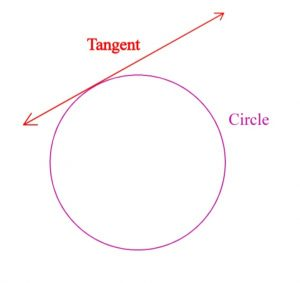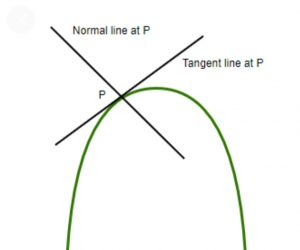Latest SSC jobs   »   Tangents   »   Tangents

# Tangents of Circle, Formula, Properties and Equation

## Tangents

Tangents: Tangent is a Latin word that means to touch. A tangent is defined as a straight line that touches the circle or ellipse at any single point and doesn’t enter the circle. In geometry, the tangent is the straight lime touches at any point of the circumference of a circle. Here we are going to discuss the meaning of tangent, tangent to a circle, theorem of a tangent, etc. So stay tuned to this article to get detailed knowledge about tangents.## Meaning of Tangents

In geometry, a tangent is defined as the straight line drawn from any single point to the circumference of a circle. It is also defined as the line which represents the slope of a curve at that particular point. A line touches the point called the point of tangency. In our daily life, we can experience a tangent easily, if we roll a ball or ring on the surface then every point of the circumference of the wheel makes the tangent.

## Tangents of Circle

A tangent is a line that passes through a point on the circumference of a circle and never enters the circle. A circle has infinite tangents. It touches the circle at only a single point.Consider an arc C and a point external to arc C is any point. A straight line drawn from that point to C touches the circumference of a circle at point P known as the point of tangency. This is an example of a tangent. The tangent is shown in the above figure clearly.

### Point of Tangency

A point of tangency is a point at which the straight line passes or touches it. In the above figure point, P is the point of tangency.

### Properties of Tangent

Following are the important properties of a tangent:

• A tangent touches or intersects the circle at any single point on its circumference.
• A tangent never enters inside the circle.
• A tangent intersects the point on the circumference at a right angle.

## Tangents Formula

In trigonometry, the tangent is the ratio of the length of the opposite side to the adjacent side of the right-angled triangle. It is also defined as the sine to cosine function of an acute angle such that the value of the cosine function is not equal to zero. Tangent is one of the important trigonometric functions from a total of six functions.

Consider a right-angled triangle ABC in which angle ABC is, AB is the hypotenuse, BC is the adjacent side and AC is the opposite side respectively.

Tanθ = Sinθ/Cosθ

In trigonometry, the tangent function is used to represent the slope of a line between the intersecting of the hypotenuse and an altitude of a right-angled triangle. In both trigonometry and geometry tangent function is used to find the slope of many objects concerning the origin.

## Applications of Tangent

There are many applications of tangent in many fields. Some important applications are listed below:

• In solving maxima and minima problems
• In curve sketching
• In distance, velocity, and acceleration problems
• Approximation function
• In solving Rated functions
• In height and distance problems
• In gradient and slope analysis

## Equations of Tangents

Consider any curve, on which a tangent line passes through a single point located at the circumference and a normal drawn to it. Then we are going to derive the equation of tangent from this.

We know that the equation of a straight line passes through any point (x0, y0) making a slope m. The equation of slope is given by

y – y0 = m (x – x0)

The slope of a tangent line to the curve f(x)=y at a origin (x0, y0) is given by

dy/dx (x0, y0) = [f’ (x0)]

Therefore the equation of the tangent (x0, y0) with a curve y=f(x) is given by

y – y0 = f ′(x0)(x – x0)

Generally the normal is perpendicular to the tangent line. Hence the slope normal to the curve f(x)=y at point (x0, y0) is given by

y-y0 = [-1/f’(x0)] (x-x0)

The above equation may be written as also

(y-y0) f’(x0) + (x-x0) = 0

Que. Find the equation of tangent if equation of a straight line to a curve is given by y=x^3+2x^2-7x+1

Solution : Given equation: y=x^3+2x^2-7x+1

Differentiating the above equation with respect to x to get gradient, dy/dx = 3x^2+4x-7

at point x=3 the gradient will be, m=3(3)^2+4×3-7, m=32

Equation of tangent in the form y=mx+c, putting m=32, x=3, and y=2 to get c

y=mx+c
2=32×3+c
c=-94

Then the equation of the tangent will be, y=mx+c, y=32x-94

## Tangents: FAQs

Que.1 Define tangent?

Ans – A tangent is a straight line that passes at any single point on the circumference of the circle that never enters inside the circle.

Que.2 What is the point of tangency?

Ans – A point on which straight line passes are termed a point of tangency.

Que.3 How many tangents can be drawn from the circle?

Ans – In the circle, infinite tangents can be drawn. There are infinite points on the circumference of a circle and infinite tangents can be drawn from it. A line is simply called a tangent line.

Que.4 Is tangent can enter inside the circle?

Ans – No, tangents can never be entered to induce the circle. It passes from a single point. If it enters inside the circle then it will be termed as a chord of the circle.

#### Congratulations!General Awareness & Science Capsule PDF